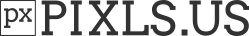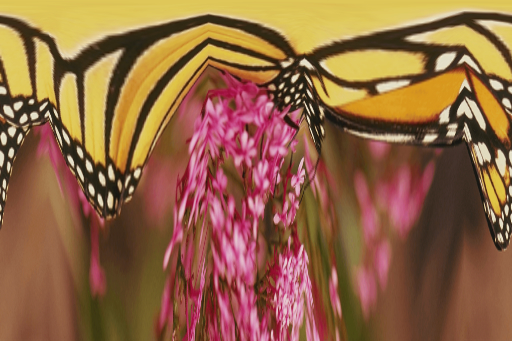# Geometry Help - Rectangular Polar Transformation (From Cartesian or To Cartesian) [SOLVED OR NEARLY SOLVED]

At the moment, I do have the rectangular polar transform almost ready after I solved some quirks, but one issue do remain - After I move the point from which the transform use as a reference for output, the result goes out of bound.

To explain what is Rectangular Polar Image

Your output is generated from reading pixels going from the direction of these line. The greater the Y-Axis, the farther away from the center it’s going to be. When Y is 1, then the output would show that pixel to be located at the bottom of the image.Treat the X line as pixel scanning, and it is what determines the angle from the center when Y is greater than 0.

Transform Input/Output

To show how the result would look like when the transform affects a image when the centerpoint is located at 0,0 - let’s use a sample image:

Target ImageTransformed ImageThe current G’MIC implementation

``````rep_polar_rectangular_transform:
skip \${1=.5},\${2=.5}
f "
begin(
point_x=\$1*w;
point_y=\$2*h;
inv_point_x=w-point_x;
inv_point_y=h-point_y;
cut_ang_s0=atan2(point_y,inv_point_x)*180/pi;
cut_ang_s1=180-atan2(point_y,point_x)*180/pi;
cut_ang_s2=180+abs(atan2(inv_point_y,point_x)*180/pi);
cut_ang_s3=360-abs(atan2(inv_point_y,inv_point_x)*180/pi);
distanceaway(value)=(
if(value==0,w-point_x?w-point_x:1,
if(value==1,h-point_y?h-point_y:1,
if(value==2,point_x?point_x:1,
if(value==3,point_y?point_y:1
);
);
);
);
);
);
surface_angle=(x/w)*360;
sur_180=surface_angle-180*floor(surface_angle/180+(10^-8));
sur_90=sur_180>90?180-sur_180:sur_180;
if(surface_angle>cut_ang_s0&&surface_angle<=cut_ang_s1,side=1, #Top#
if(surface_angle>cut_ang_s1&&surface_angle<=cut_ang_s2,side=2, #Right#
if(surface_angle>cut_ang_s2&&surface_angle<=cut_ang_s3,side=3, #Bottom#
side=0; #Left#
);
);
);
orientation=side%2;
mdist=orientation?90-sur_90:sur_90;
mdist=1/cos((mdist/180)*pi);
dix=(point_x+cos((surface_angle/180)*pi)*distanceaway(side)*mdist*y/h)*((w-1)/w);
diy=(point_y+sin((surface_angle/180)*pi)*distanceaway(side)*mdist*y/h)*((h-1)/h);
i(dix,diy,z,c,1);
"
``````

That being said, what exactly I’m doing wrong when using point location change. Why it goes out of bound?

EDIT: I fixed it for \$1. Not for \$2. That means result will be correct with \$2=.5. If not, then result will be incorrect.

EDIT: I finally determine the cause of my issue has to do with the mdist line. I would need to figure how to create a gradient based on angle found. After that, this command would work.

Solved or almost solved!

Last post for this thread. Decided to not to try to solve micro-shifting, and call it a day with these command. You can consider it solved, but if micro-shifting is a big issue on preserved details, then consider it nearly solved, but I can’t do it.

``````#@cli rep_polrectrans_inv : eq. to 'rep_polar_rectangular_transform_inverse'. : (+)
rep_polrectrans_inv: rep_polar_rectangular_transform_inverse \$*
#@cli rep_polar_rectangular_transform_inverse : -1>=_xpos<=1,-1>=_ypos<=1,_from_preserved_details={ 0=false | 1=true }
#@cli : Transform rectangular polar images into cartesian coordinates.
#@cli : (eq. to 'rep_polrectrans_inv').\n
#@cli : Warning: Without preservation of details, artifacts is a lot more visible.
#@cli : Default values: '_xpos=0','_ypos=0','_preserve_details=1'
rep_polar_rectangular_transform_inverse:
skip \${1=0},\${2=0},\${3=1}
if \$1<-1||\$1>1 error "(\$1>=-1&&\$1<=1)=0" fi
if \$2<-1||\$2>1 error "(\$2>=-1&&\$2<=1)=0" fi
if \$3
repeat \$! l[\$>]
ov={[im,iM]}
{w/2-h/2},{h/2},{d},{s},"
begin(
ww=w#0;
hh=h#0;
ox=ww/2-hh/2;
oy=hh/2;
sd=max(ww,hh)/min(ww,hh);
sxf=ox<oy?sd:1;
syf=ox<oy?1:sd;
cx=.5+\$1*.5;
cy=.5+\$2*.5;
px=cx*ww;
py=(1-cy)*hh;
sxl=(ww/2)/px;
sxr=(ww/2)/(ww-px);
syt=(hh/2)/py;
syb=(hh/2)/(hh-py);
);
xx=(x/(w-1))*ww;xx+=(xx/(ww-1)-.5)*-1;
yy=(y/h)*hh;
xl=-1+(xx/ww)*2*sxl;
xr=1-(1-xx/(ww-1))*2*sxr;
yt=-1+(yy/hh)*2*syt;
yb=1-(1-yy/(hh-1))*2*syb;
nxx=xx>px?xr:xl;
nyy=yy>py?yb:yt;
ay=max(abs(nxx),abs(nyy));
ax=(atan2((yy/hh-(1-cy))*syf,(xx/ww-cx)*sxf)+pi)/(2*pi);
i(#0,ax*ww,ay*hh,z,c,1,1);
"
n \$ov
k.
endl done
else
ov={[im,iM]}
f "
begin(
sd=(max(w,h)/min(w,h));
sx=w>h?sd:1;
sy=w>h?1:sd;
ww=w-1;
hh=h-1;
cx=.5+\$1*.5;
cy=.5+\$2*.5;
px=cx*w;
py=(1-cy)*h;
sxl=(w/2)/px;
sxr=(w/2)/(w-px);
syt=(h/2)/py;
syb=(h/2)/(h-py);
);
xl=-1+(x/w)*2*sxl;
xr=1-(1-x/w)*2*sxr;
yt=-1+(y/h)*2*syt;
yb=1-(1-y/h)*2*syb;
xx=x>px?xr:xl;
yy=y>py?yb:yt;
ay=max(abs(xx),abs(yy));
xx=(x/w)-cx;
yy=(y/h)-(1-cy);
ax=(atan2(yy*sy,xx*sx)+pi)/(2*pi);
i(ax*ww,ay*hh,z,c,2,1);
"
n \$ov
fi
#@cli rep_polrectrans : eq. to 'rep_polar_rectangular_transform'. : (+)
rep_polrectrans: rep_polar_rectangular_transform \$*
#@cli rep_polar_rectangular_transform : -1>=_xpos<=1,-1>=_ypos<=1,_preserve_details={ 0=false | 1=true }
#@cli : Transform image into rectangular polar coordinates.
#@cli : (eq. to 'rep_polrectrans').\n
#@cli : Default values: '_xpos=0','_ypos=0','_preserve_details=1'
rep_polar_rectangular_transform:
skip \${1=0},\${2=0},\${3=1}
if \$1<-1||\$1>1 error "(\$1>=-1&&\$1<=1)=0" fi
if \$2<-1||\$2>1 error "(\$2>=-1&&\$2<=1)=0" fi
repeat \$! l[\$>]
ov={[im,iM]}
if \$3 r {(w+h)*2},200%,100%,100%,1 fi #Resize to perimeter for width and double the height for height to preserve all the details in the original image using nearest-neighbor#
f "
begin(
point_x=((\$1*-1)*.5+.5)*w;
point_y=(\$2*.5+.5)*h;
inv_point_x=w-point_x;
inv_point_y=h-point_y;
cut_ang_s0=abs(atan2(inv_point_y,inv_point_x)*180/pi); #Find angle requirement to meet side#
cut_ang_s1=180-abs(atan2(inv_point_y,point_x)*180/pi); #Find angle requirement to meet side#
cut_ang_s2=180+abs(atan2(point_y,point_x)*180/pi);     #Find angle requirement to meet side#
cut_ang_s3=360-abs(atan2(point_y,inv_point_x)*180/pi); #Find angle requirement to meet side#
distanceaway(value)=(
if(value==0,w-point_x, #Left#
if(value==1,h-point_y, #Top#
if(value==2,point_x,   #Right#
if(value==3,point_y    #Bottom#
);
);
);
);
);
);
surface_angle=(x/w)*360; #Convert into angle surface using x-coordinate#
if(surface_angle>cut_ang_s0&&surface_angle<=cut_ang_s1,side=1, #Top#
if(surface_angle>cut_ang_s1&&surface_angle<=cut_ang_s2,side=2, #Right#
if(surface_angle>cut_ang_s2&&surface_angle<=cut_ang_s3,side=3, #Bottom#
side=0; #Left#
);
);
);
mdist=abs(side%2?1/sin((surface_angle/180)*pi):1/cos((surface_angle/180)*pi));    #Find multiplier to distance based on angle to edge boundary#
dix=(point_x+cos((surface_angle/180)*pi)*distanceaway(side)*mdist*y/h)*((w-1)/w); #x-coordinate#
diy=(point_y+sin((surface_angle/180)*pi)*distanceaway(side)*mdist*y/h)*((h-1)/h); #y-coordinate#
i(w-(dix+1),h-(diy+1),z,c,2); #Output using pixel coordinate with linear interpolation#
"
cut \$ov
endl done
``````